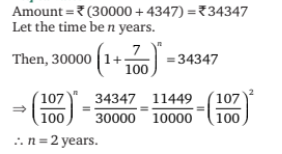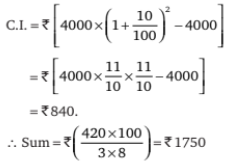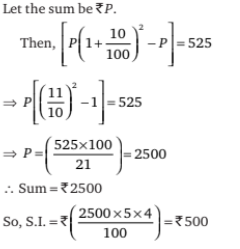# Mathematical Aptitude - Interest

>>>>>>>>Interest

• A

2• B

2 1/2• C

3• D

4• Option : A
• Explanation :• A

Rs 1550• B

Rs 1650• C

Rs 1750• D

Rs 2000• Option : C
• Explanation :• A

Rs 400• B

Rs 500• C

Rs 600• D

Rs 800• Option : B
• Explanation :• A

7.85%• B

9.41%• C

6.66%• D

4.21%• Option : C
• Explanation : Let the principle be P.
As the amount doubles itself the interest is P too.
So P = P × r × 15/100
⇒ r = 100/15 = 20/3% = 6.66%

• A

Rs 1800• B

Rs 2000• C

Rs 1400• D

Rs 1250• Option : D
• Explanation : 2500 in 5th year and 3000 in 7th year.
So in between 2 years, Rs 500 has increased.
⇒ For a year 500/2 = 250
So, per year it is increasing Rs 250, then in 5 years
⇒ 250 × 5 = 1250
Hence, the initial amount must be 2500 – 1250
= Rs 1250.

## Description

• Simple Interest Questions can be used to give quizzes by any candidate who is preparing for UGC NET Paper 1
• This Simple Interest Questions section will help you test your analytical skills in a tricky method, thereby giving you an edge over other students
• All candidates who have to appear for the Kendriya Vidyalaya Entrance exam can also refer to this mcq section.
• You can also get access to the Simple Interest MCQ ebook.
• Simple Interest Questions can be used in the preparation of JRF, CSIR, and various other exams.
• You can also download pdf for these Simple Interest multiple-choice questions Answers.
• This Simple Interest Multiple Choice Questions Answers section can also be used for the preparation of various competitive exams like UGC NET, GATE, PSU, IES, and many more.
• Simple Interest Questions can be used to gain a credit score in various undergraduate and postgraduate courses like Bcom, Mcom and more

Related Quiz.
Interest